Next: Two Examples Up: Robust Estimation Previous: M-estimators

## Least Median of Squares

The least-median-of-squares (LMedS) method estimates the parameters by solving the nonlinear minimization problem: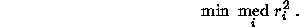That is, the estimator must yield the smallest value for the median of squared residuals computed for the entire data set. It turns out that this method is very robust to false matches as well as outliers due to bad localization. Unlike the M-estimators, however, the LMedS problem cannot be reduced to a weighted least-squares problem. It is probably impossible to write down a straightforward formula for the LMedS estimator. It must be solved by a search in the space of possible estimates generated from the data. Since this space is too large, only a randomly chosen subset of data can be analyzed. The algorithm which we describe below for robustly estimating a conic follows that structured in [22, Chap.,], as outlined below.

Given n points: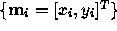.

1. A Monte Carlo type technique is used to draw m random subsamples of p different points. For the problem at hand, we select five (p=5) points because we need at least five points to define a conic.
2. For each subsample, indexed by J, we use any of the techniques described in Sect.4 to compute the conic parameters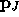. (Which technique is used is not important because an exact solution is possible for five different points.)
3. For each, we can determine the median of the squared residuals, denoted by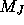, with respect to the whole set of points,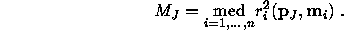Here, we have a number of choices for, the residual of the ipoint with respect to the conic. Depending on the demanding precision, computation requirement, etc., one can use the algebraic distance, the Euclidean distance, or the gradient weighted distance.

4. We retain the estimatefor whichis minimal among all m's.
The question now is: How do we determine m? A subsample is ``good'' if it consists of p good data points. Assuming that the whole set of points may contain up to a fractionof outliers, the probability that at least one of the m subsamples is good is given by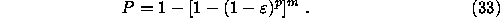By requiring that P must be near 1, one can determine m for given values of p and: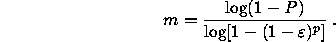In our implementation, we assume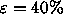and require P=0.99, thus m=57. Note that the algorithm can be speeded up considerably by means of parallel computing, because the processing for each subsample can be done independently.

As noted in , the LMedS efficiency is poor in the presence of Gaussian noise. The efficiency of a method is defined as the ratio between the lowest achievable variance for the estimated parameters and the actual variance provided by the given method. To compensate for this deficiency, we further carry out a weighted least-squares procedure. The robust standard deviation estimate is given bywhereis the minimal median. The constant 1.4826 is a coefficient to achieve the same efficiency as a least-squares in the presence of only Gaussian noise; 5/(n-p) is to compensate the effect of a small set of data. The reader is referred to [22, page 202,] for the details of these magic numbers. Based on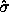, we can assign a weight for each correspondence: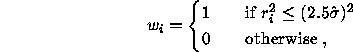whereis the residual of the ipoint with respect to the conic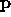. The correspondences having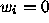are outliers and should not be further taken into account. The conicis finally estimated by solving the weighted least-squares problem: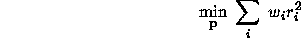using one of the numerous techniques described before. We have thus robustly estimated the conic because outliers have been detected and discarded by the LMedS method.

As said previously, computational efficiency of the LMedS method can be achieved by applying a Monte-Carlo type technique. However, the five points of a subsample thus generated may be very close to each other. Such a situation should be avoided because the estimation of the conic from such points is highly instable and the result is useless. It is a waste of time to evaluate such a subsample. In order to achieve higher stability and efficiency, we develop a regularly random selection method based on bucketing techniques, which works as follows. We first calculate the min and max of the coordinates of the points in the first image. The region is then evenly divided intobuckets (see fig:bucketing; in our implementation, b=8). To each bucket is attached a set of points, and indirectly a set of matches, which fall in it. The buckets having no matches attached are excluded. To generate a subsample of 5 points, we first randomly select 5 mutually different buckets, and then randomly choose one match in each selected bucket.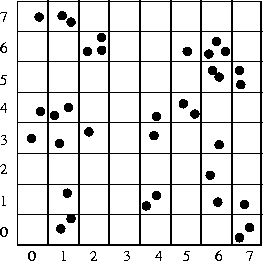Figure 5: Illustration of a bucketing technique

One question remains: How many subsamples are required? If we assume that bad points are uniformly distributed in space, and if each bucket has the same number of points and the random selection is uniform, the formula (33) still holds. However, the number of points in one bucket may be quite different from that in another. As a result, a point belonging to a bucket having fewer points has a higher probability to be selected. It is thus preferred that a bucket having many points has a higher probability to be selected than a bucket having few points, in order that each point has almost the same probability to be selected. This can be realized by the following procedure. If we have in total l buckets, we divide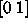into l intervals such that the width of the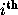interval is equal to, where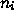is the number of points attached to thebucket (see fig:prob-mapping). During the bucket selection procedure, a number, produced by auniform random generator, falling in theinterval implies that thebucket is selected.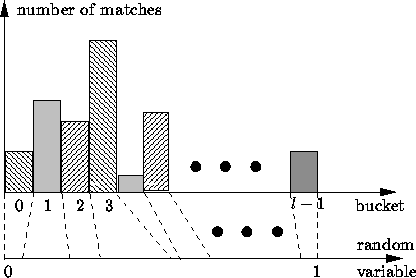Figure 6: Interval and bucket mapping

We have applied this technique to matching between two uncalibrated images . Given two uncalibrated images, the only available geometric constraint is the epipolar constraint. The idea underlying our approach is to use classical techniques (correlation and relaxation methods in our particular implementation) to find an initial set of matches, and then use the Least Median of Squares (LMedS) to discard false matches in this set. The epipolar geometry can then be accurately estimated using a meaningful image criterion. More matches are eventually found, as in stereo matching, by using the recovered epipolar geometry.Next: Two Examples Up: Robust Estimation Previous: M-estimators

Zhengyou Zhang
Thu Feb 8 11:42:20 MET 1996